login  home  contents  what's new  discussion  bug reports help  links  subscribe  changes  refresh  edit

A generic way to define function that cache their values.

)abbrev domain CACHEDF CachedFunction
++ Author: Ralf Hemmecke
++ Date Created: 2009
++ Date Last Updated: Oct 27, 2009
++ Basic Operations:
++ Related Domains: Table, AssociationList
++ Also See:
++ AMS Classifications:
++ Keywords:
++ Examples:
++ References:
++ Description:
++   This domain is a domain of functions with associated cache.
CachedFunction(A: SetCategory, B: SetCategory): Exports == Implementation where
Exports ==> with
function: % -> (A -> B)
cachedFunction: (A -> B) -> %
apply: (%, A) -> B
recursiveDefine: (%, f: A -> B) -> %
Rep := Record(cache: Table(A, B), fun: A -> B);
function(x: %): A -> B == x.fun
cachedFunction(f: A -> B): % == [empty()$Table(A, B), f] apply(x: %, a: A): B == c := x.cache u: Union(B, "failed") := search(a, c) if u case B then u :: B else f: A -> B := x.fun c.a := f(a) recursiveDefine(x: %, f: A -> B): % == x.fun := f; x spad  Compiling FriCAS source code from file /var/lib/zope2.10/instance/axiom-wiki/var/LatexWiki/8303643491408128431-25px001.spad using old system compiler. CACHEDF abbreviates domain CachedFunction ------------------------------------------------------------------------ initializing NRLIB CACHEDF for CachedFunction compiling into NRLIB CACHEDF compiling exported function :$ -> A -> B
CACHEDF;function;$M;1 is replaced by QCDR Time: 0 SEC. compiling exported cachedFunction : A -> B ->$
Time: 0 SEC.
compiling exported apply : ($,A) -> B Time: 0.01 SEC. compiling exported recursiveDefine : ($,A -> B) -> $Time: 0 SEC. (time taken in buildFunctor: 0) ;;; *** |CachedFunction| REDEFINED ;;; *** |CachedFunction| REDEFINED Time: 0 SEC. Warnings:  function: fun has no value  apply: cache has no value  apply: fun has no value  recursiveDefine: fun has no value Cumulative Statistics for Constructor CachedFunction Time: 0.01 seconds finalizing NRLIB CACHEDF Processing CachedFunction for Browser database: --------constructor--------- --->-->CachedFunction((function ((Mapping B A) %))): Not documented!!!! --->-->CachedFunction((cachedFunction (% (Mapping B A)))): Not documented!!!! --->-->CachedFunction((apply (B % A))): Not documented!!!! --->-->CachedFunction((recursiveDefine (% % (Mapping B A)))): Not documented!!!! ; compiling file "/var/aw/var/LatexWiki/CACHEDF.NRLIB/CACHEDF.lsp" (written 04 APR 2022 07:27:27 PM): ; /var/aw/var/LatexWiki/CACHEDF.NRLIB/CACHEDF.fasl written ; compilation finished in 0:00:00.028 ------------------------------------------------------------------------ CachedFunction is now explicitly exposed in frame initial CachedFunction will be automatically loaded when needed from /var/aw/var/LatexWiki/CACHEDF.NRLIB/CACHEDF fricas )set message time on I := Integer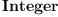(1) Type: Type fricas Time: 0.02 (OT) = 0.02 sec f(n:I): I ==1 Function declaration f : Integer -> Integer has been added to workspace. Type: Void fricas Time: 0 sec fib: CachedFunction(I,I) := cachedFunction(f) fricas Compiling function f with type Integer -> Integer LISP output: (#<HASH-TABLE :TEST EQUAL :COUNT 0 {10051A83F3}> #<FUNCTION |*1;f;1;initial|>) Type: CachedFunction?(Integer,Integer) fricas Time: 0.01 (OT) = 0.01 sec recursiveDefine(fib,(n:I):I+->if n<2 then 1 else fib(n-1) + fib(n-2)) LISP output: (#<HASH-TABLE :TEST EQUAL :COUNT 0 {10051A83F3}> #<FUNCTION |*1;anonymousFunction;0;initial;internal|>) Type: CachedFunction?(Integer,Integer) fricas Time: 0.01 (IN) = 0.01 sec fib 40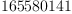(2) Type: PositiveInteger? fricas Time: 0.01 (IN) = 0.01 sec g(n:I): I == if n<2 then 1 else g(n-1)+g(n-2) Function declaration g : Integer -> Integer has been added to workspace. Type: Void fricas Time: 0 sec g 40 fricas Compiling function g with type Integer -> Integer(3) Type: PositiveInteger? fricas Time: 3.32 (EV) = 3.32 sec h: I -> I := function fib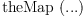(4) Type: (Integer -> Integer) fricas Time: 0 sec h 40(5) Type: PositiveInteger? fricas Time: 0 sec fricas )set message time off ## Caching a function with more than one argument We need to combine more than one domain into a single domain in a universal manner like a cross-product. There are seveal ways to do this in Axiom including List, Record, Product and DirectProduct. For example: fricas -- combine two domain in one using Record Pair:=Record(x1:Integer,x2:Integer)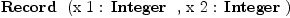(6) Type: Type fricas pair(x:Integer,y:Integer):Pair == [x,y] Function declaration pair : (Integer, Integer) -> Record(x1: Integer ,x2: Integer) has been added to workspace. Type: Void fricas -- gcd2(arg:Pair):Integer == gcd(arg.x1,arg.x2) Function declaration gcd2 : Record(x1: Integer,x2: Integer) -> Integer has been added to workspace. Type: Void fricas gcd2cached:CachedFunction(Pair,Integer):=cachedFunction gcd2 fricas Compiling function gcd2 with type Record(x1: Integer,x2: Integer) -> Integer LISP output: (() #<FUNCTION |*1;gcd2;1;initial|>) Type: CachedFunction?(Record(x1: Integer,x2: Integer),Integer) fricas gcdCached(x1:Integer,x2:Integer):Integer == gcd2cached pair(x1,x2) Function declaration gcdCached : (Integer, Integer) -> Integer has been added to workspace. Type: Void fricas )set message time on for i in 1..100 repeat for j in 1..100 repeat gcd(i,j) Type: Void fricas Time: 0.33 (EV) = 0.33 sec for i in 1..100 repeat for j in 1..100 repeat gcdCached(i,j) fricas Compiling function pair with type (Integer, Integer) -> Record(x1: Integer,x2: Integer) fricas Compiling function gcdCached with type (Integer, Integer) -> Integer Type: Void fricas Time: 0.01 (IN) + 7.09 (EV) = 7.10 sec fricas )set message time off fricas testhash() == for i in 1..100 repeat for j in 1..100 repeat if gcdCached(i,j)~=gcd(i,j) then error "bad hash!" "good" Type: Void fricas testhash() fricas Compiling function testhash with type () -> String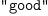(7) Type: String I was rather disappointed with the performance of the cache! So I took a look at the source code for Table and it seems that Table only uses an efficient hashing method if hashable(Key)$Lisp is true, where Key is the first domain of Table(Key,Entry). This is true for Integer and String but not Record(x1:Integer,x2:Integer) or even List(Integer).

fricas
hashable(Integer)$Lisp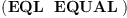(8) Type: SExpression? fricas hashable(String)$Lisp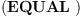(9)
Type: SExpression?
fricas
hashable(Record(x1:Integer,x2:Integer))$Lisp Compiled code for gcd2 has been cleared.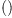(10) Type: SExpression? fricas hashable(List Integer)$Lisp(11)
Type: SExpression?

But as far as I can see the implementation of List and Record in Axiom do satisfy the requirements for the use of HashTable?:

  ++   This creates a \spadtype{HashTable} if equal for the Key
++   domain is consistent with Lisp EQUAL otherwise an


if their component domains do. So I think it would be a good idea to make the hashable function in 'spad.lisp':

  (defun |knownEqualPred| (dom)
(let ((fun (|compiledLookup| '= '((|Boolean|) ) dom)))
(if fun (get (bpiname (car fun)) '|SPADreplace|)
nil)))

(defun |hashable| (dom)
(memq (|knownEqualPred| dom)
#-Lucid '(EQ EQL EQUAL)
#+Lucid '(EQ EQL EQUAL EQUALP)
))


a little smarter. This test only seems to check if the compiler was able to optimize equality to a lisp operator. I suppose we could improve the compiler but even failing that, there should be a way to recognize it at this level. I think it's something like having the Canonical property "all the way down".

fricas
)clear completely
All user variables and function definitions have been cleared.
All )browse facility databases have been cleared.
Internally cached functions and constructors have been cleared.
)clear completely is finished.

We can test this for now by using HashTable? explicitly and just assuming that that domain A satisfies the requirement:

)abbrev domain CACHEDF CachedFunction
CachedFunction(A: SetCategory, B: SetCategory): Exports == Implementation where
Exports ==> with
function: % -> (A -> B)
cachedFunction: (A -> B) -> %
apply: (%, A) -> B
recursiveDefine: (%, f: A -> B) -> %
Rep := Record(cache: HashTable(A, B, "UEQUAL"), fun: A -> B);
function(x: %): A -> B == x.fun
cachedFunction(f: A -> B): % == [empty()$HashTable(A, B, "UEQUAL"), f] apply(x: %, a: A): B == c := x.cache u: Union(B, "failed") := search(a, c) if u case B then u :: B else f: A -> B := x.fun c.a := f(a) recursiveDefine(x: %, f: A -> B): % == x.fun := f; x spad  Compiling FriCAS source code from file /var/lib/zope2.10/instance/axiom-wiki/var/LatexWiki/6894297888104578709-25px008.spad using old system compiler. CACHEDF abbreviates domain CachedFunction ------------------------------------------------------------------------ initializing NRLIB CACHEDF for CachedFunction compiling into NRLIB CACHEDF Local variable Rep type redefined: (Join (SetCategory) (CATEGORY domain (SIGNATURE construct ((Record (: cache (HashTable A B UEQUAL)) (: fun (Mapping B A))) (HashTable A B UEQUAL) (Mapping B A))) (SIGNATURE ~= ((Boolean) (Record (: cache (HashTable A B UEQUAL)) (: fun (Mapping B A))) (Record (: cache (HashTable A B UEQUAL)) (: fun (Mapping B A))))) (SIGNATURE coerce ((OutputForm) (Record (: cache (HashTable A B UEQUAL)) (: fun (Mapping B A))))) (SIGNATURE elt ((HashTable A B UEQUAL) (Record (: cache (HashTable A B UEQUAL)) (: fun (Mapping B A))) cache)) (SIGNATURE elt ((Mapping B A) (Record (: cache (HashTable A B UEQUAL)) (: fun (Mapping B A))) fun)) (SIGNATURE setelt! ((HashTable A B UEQUAL) (Record (: cache (HashTable A B UEQUAL)) (: fun (Mapping B A))) cache (HashTable A B UEQUAL))) (SIGNATURE setelt! ((Mapping B A) (Record (: cache (HashTable A B UEQUAL)) (: fun (Mapping B A))) fun (Mapping B A))) (SIGNATURE copy ((Record (: cache (HashTable A B UEQUAL)) (: fun (Mapping B A))) (Record (: cache (HashTable A B UEQUAL)) (: fun (Mapping B A))))))) to (Join (SetCategory) (CATEGORY domain (SIGNATURE construct ((Record (: cache (Table A B)) (: fun (Mapping B A))) (Table A B) (Mapping B A))) (SIGNATURE ~= ((Boolean) (Record (: cache (Table A B)) (: fun (Mapping B A))) (Record (: cache (Table A B)) (: fun (Mapping B A))))) (SIGNATURE coerce ((OutputForm) (Record (: cache (Table A B)) (: fun (Mapping B A))))) (SIGNATURE elt ((Table A B) (Record (: cache (Table A B)) (: fun (Mapping B A))) cache)) (SIGNATURE elt ((Mapping B A) (Record (: cache (Table A B)) (: fun (Mapping B A))) fun)) (SIGNATURE setelt! ((Table A B) (Record (: cache (Table A B)) (: fun (Mapping B A))) cache (Table A B))) (SIGNATURE setelt! ((Mapping B A) (Record (: cache (Table A B)) (: fun (Mapping B A))) fun (Mapping B A))) (SIGNATURE copy ((Record (: cache (Table A B)) (: fun (Mapping B A))) (Record (: cache (Table A B)) (: fun (Mapping B A))))))) compiling exported function :$ -> A -> B
CACHEDF;function;$M;1 is replaced by QCDR ;;; *** |CACHEDF;function;$M;1| REDEFINED
Time: 0 SEC.
compiling exported cachedFunction : A -> B -> $;;; *** |CACHEDF;cachedFunction;M$;2| REDEFINED
Time: 0.01 SEC.
compiling exported apply : ($,A) -> B ;;; *** |CACHEDF;apply;$AB;3| REDEFINED
Time: 0 SEC.
compiling exported recursiveDefine : ($,A -> B) ->$
;;;     ***       |CACHEDF;recursiveDefine;$M$;4| REDEFINED
Time: 0 SEC.
(time taken in buildFunctor:  0)
;;;     ***       |CachedFunction| REDEFINED
;;;     ***       |CachedFunction| REDEFINED
Time: 0 SEC.
Warnings:
 function:  fun has no value
 apply:  cache has no value
 apply:  fun has no value
 recursiveDefine:  fun has no value
Cumulative Statistics for Constructor CachedFunction
Time: 0.01 seconds
finalizing NRLIB CACHEDF
Processing CachedFunction for Browser database:
--->/var/lib/zope2.10/instance/axiom-wiki/var/LatexWiki/8303643491408128431-25px001.spad-->CachedFunction((function ((Mapping B A) %))): Not documented!!!!
--->/var/lib/zope2.10/instance/axiom-wiki/var/LatexWiki/8303643491408128431-25px001.spad-->CachedFunction((cachedFunction (% (Mapping B A)))): Not documented!!!!
--->/var/lib/zope2.10/instance/axiom-wiki/var/LatexWiki/8303643491408128431-25px001.spad-->CachedFunction((apply (B % A))): Not documented!!!!
--->/var/lib/zope2.10/instance/axiom-wiki/var/LatexWiki/8303643491408128431-25px001.spad-->CachedFunction((recursiveDefine (% % (Mapping B A)))): Not documented!!!!
; compiling file "/var/aw/var/LatexWiki/CACHEDF.NRLIB/CACHEDF.lsp" (written 04 APR 2022 07:27:42 PM):
; /var/aw/var/LatexWiki/CACHEDF.NRLIB/CACHEDF.fasl written
; compilation finished in 0:00:00.025
------------------------------------------------------------------------
CachedFunction is now explicitly exposed in frame initial
CachedFunction will be automatically loaded when needed from
/var/aw/var/LatexWiki/CACHEDF.NRLIB/CACHEDF

Now let's try that again.

fricas
-- combine two domain in one
Pair:=Record(x1:Integer,x2:Integer)(12)
Type: Type
fricas
pair(x:Integer,y:Integer):Pair == [x,y]
Function declaration pair : (Integer, Integer) -> Record(x1: Integer
,x2: Integer) has been added to workspace.
Type: Void
fricas
--
gcd2(arg:Pair):Integer == gcd(arg.x1,arg.x2)
Function declaration gcd2 : Record(x1: Integer,x2: Integer) ->
Integer has been added to workspace.
Type: Void
fricas
gcd2cached:CachedFunction(Pair,Integer):=cachedFunction gcd2
fricas
Compiling function gcd2 with type Record(x1: Integer,x2: Integer)
-> Integer
LISP output:
(#<HASH-TABLE :TEST EQUAL :COUNT 0 {1004DE5253}> #<FUNCTION |*1;gcd2;1;initial|>)
Type: CachedFunction?(Record(x1: Integer,x2: Integer),Integer)
fricas
gcdCached(x1:Integer,x2:Integer):Integer == gcd2cached pair(x1,x2)
Function declaration gcdCached : (Integer, Integer) -> Integer has
been added to workspace.
Type: Void

fricas
)set message time on
for i in 1..100 repeat for j in 1..100 repeat gcd(i,j)
Type: Void
fricas
Time: 0.28 (EV) = 0.28 sec
for i in 1..100 repeat for j in 1..100 repeat gcdCached(i,j)
fricas
Compiling function pair with type (Integer, Integer) -> Record(x1:
Integer,x2: Integer)
fricas
Compiling function gcdCached with type (Integer, Integer) -> Integer
Type: Void
fricas
Time: 0.01 (IN) + 0.22 (EV) = 0.23 sec
fricas
)set message time off

fricas
testhash() ==
for i in 1..100 repeat for j in 1..100 repeat
if gcdCached(i,j)~=gcd(i,j) then error "bad hash!"
"good"
Type: Void
fricas
testhash()
fricas
Compiling function testhash with type () -> String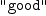(13)
Type: String

perhaps recursiveDefine not necessary --Bill Page, Wed, 28 Oct 2009 22:06:18 -0700 reply
fricas
)set message time on

fricas
)lib CACHEDF
CachedFunction is already explicitly exposed in frame initial
CachedFunction will be automatically loaded when needed from
/var/aw/var/LatexWiki/CACHEDF.NRLIB/CACHEDF
fib2: CachedFunction(INT,INT):= cachedFunction (n+->
if n<2 then 1 else fib2(n-1)+fib2(n-2)
)
LISP output:
(#<HASH-TABLE :TEST EQUAL :COUNT 0 {1007D93353}> #<FUNCTION |*1;anonymousFunction;1;initial;internal|>)
Type: CachedFunction?(Integer,Integer)
fricas
Time: 0.01 (OT) = 0.01 sec
fib2 40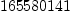(14)
Type: PositiveInteger?
fricas
Time: 0.01 (IN) = 0.01 sec

Looks like the time difference (if any) is not significant.

 Subject:   Be Bold !! ( 14 subscribers )